# States of Matter NEET Questions : NEET Previous year questions

1. Dipole-induced dipole interactions are present in which of the following pairs?   (NEET 2013)

(a) HCl and He atoms       (b) SiF4 and He atoms

(c) H2O and alcohol          (d) Cl2 and CCl4

### Redox Reactions NEET Previous year Questions with Answer key

6. A mixture of N2 and AR gases in a cylinder contains 7 g of N2 and 8 g of Ar. If the total pressure of the mixture of the gases in the cylinder is 27 bar, the

Partial pressure of N2 is  (NEET 2020)

[Use atomic masses (in g mol –1) : N = 14, AR = 40]

(a) 9 bar                           (b) 12 bar

(c) 15 bar                         (d) 18 bar.

7.
The volume occupied by 1.8 g of water vapour at 374°C and 1 bar pressure will
be (NEET 2019)

[Use R = 0.083 bar L K–1 mol–1]

(a) 96.66 L                      (b) 55.87 L

(c) 3.10 L                       (d) 5.37 L

8. Equal moles of hydrogen and oxygen gases are placed in a container with a pin-hole through which both can escape. What fraction of the oxygen escapes?

In the time required for one-half of the hydrogen to escape?       (NEET 2016)

(a) 3/8                            (b) 1/2

(c) 1/8                            (d) 1/4

9. What is the density of N2 gas at 227°C and 5.00 atm pressure? (R = 0.082 L atm K–1mol–1)                                                                                     (NEET 2013)

(a) 1.40 g/mL                (b) 2.81 g/mL

(c) 3.41 g/mL                (d) 0.29 g/Ml

10. 50 mL of each gas A and of gas B takes 150 and 200 seconds respectively for effusing through a pin hole under the similar conditions. If molecular mass of gas B is 36, the molecular mass of gas A will be                                    (2012)

(a) 96                               (b) 12

(c) 32                               (d) 64

11. A certain gas takes three times as long to effuse out as helium. Its molecular mass will be                                                                 (2012)

(a) 27 u                      (b) 36 u

(c) 64 u                      (d) 9 u

12. Two gases A and B having the same volume diffuse through a porous partition in 20 and 10 seconds respectively. The molecular mass of A is 49 u.

Molecular mass of B will be                                                        (2011)

(a) 50.00 u                        (b) 12.25 u

(c) 6.50 u                          (d) 25.00 u

13. A gaseous mixture was prepared by taking equal moles of CO and N2. If the total pressure of the mixture was found 1 atmosphere, the partial pressure of the nitrogen (N ) in the mixture is                                                           (2011)

(a) 0.5 atm

(c) 0.9 atm

(b) 0.8 atm

(d) 1 atm

14. A bubble of air is underwater at temperature 15°Cand the pressure 1.5 bar. If the bubble rises to the surface where the temperature is 25°C and the pressure is 1.0 bar, what will happen to the volume of the bubble?                 (2011)

(a) Volume will become greater by a factor of 1.6.

(b) Volume will become greater by a factor of 1.1.

(c) Volume will become smaller by a factor of 0.70.

(d) Volume will become greater by a factor of 2.5

15. The pressure exerted by 6.0 g of methane gas in a 0.03 m3 vessel at 129°C is (Atomic masses:                                                                                (Mains 2010)

C = 12.01, H = 1.01 and R = 8.314 J K–1 mol–1)

(a) 215216 Pa                            (b) 13409 Pa

(c) 41648 Pa                              (d) 31684 Pa

25. By what factor does the average velocity of a gaseous molecule increase when the temperature (in Kelvin) is doubled?                                                  (2011)

(a) 2.0                          (b) 2.8

(c) 4.0                           (d) 1.4

30. The energy absorbed by each molecule (A2) of a substance is 4.4 × 10–19 J and bond energy per molecule is 4.0 × 10–19 J. The kinetic energy of the

Molecule per atom will be                                                            (2009)

(a) 2.2 × 10–19 J                 (b) 2.0 × 10–19 J

(c) 4.0 × 10–20 J                (d) 2.0 × 10–20 J

31. If a gas expands at constant temperature, it indicates that                 (2008)

(a) Kinetic energy of molecules remains the same

(b) Number of the molecules of gas increases

(c) Kinetic energy of molecules decreases

(d) Pressure of the gas increases.

38. A gas at 350 K and 15 bar has molar volume 20 percent smaller than that for an ideal gas under the same conditions. The correct option about the gas and its compressibility factor (Z) is                                                      (NEET 2019)

(a) Z < 1 and repulsive forces are dominant

(b) Z > 1 and attractive forces are dominant

(c) Z > 1 and repulsive forces are dominant

(d) Z < 1 and attractive forces are dominant.

39. A gas such as carbon monoxide would be most likely to obey the ideal gas law at (2015)

(a) Low temperatures and high pressures

(b) High temperatures and high pressures

(c) Low temperatures and low pressures

(d) High temperatures and low pressures

40. Maximum deviation from ideal gas is expected from             (NEET 2013)

(a) CH4(g)                         (b) NH3(g)

(c) H2(g)                              (d) N2(g)

41.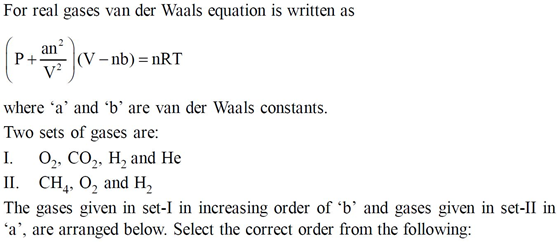(2012)

(a) (I) He < H2 < CO2 < O2 (II) CH4 > H2 > O2

(b) (I) O2 < He < H2 < CO2 (II) H2 > O2 > CH4

(c) (I) H2 < He < O2 < CO2 (II) CH4 > O2 > H2

(d) (I) H2 < O2 < He < CO2 (II) O2 > CH4 > H2

46. Given van der Waals’ constant for NH3, H2, O2 and CO2 are respectively 4.17, 0.244, 1.36 and 3.59, which one of the following gases is most easily liquefied?       (NEET 2018)

(a) NH3                                           (b) H2

(c) O2                                              (d) CO2

48. The beans are cooked earlier in pressure cooker because                 (2011)

(a) Boiling point increases with increasing pressure

(b) Boiling point decreases with increasing pressure

(c) Extra pressure of pressure cooker softens the

(d) Internal energy is not lost while cooking in pressure cooker.

71. Choose the correct option for graphical representation of Boyle’s law, which
shows a graph of pressure vs. volume of a gas at di‑erent temperatures:  (2021)

(a)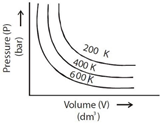(b)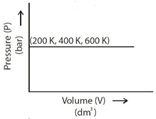(c)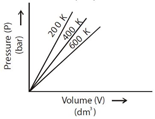(d)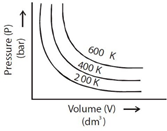87. Choose the correct option for the total pressure (in atm.) in a mixture of 4gO2 and 2gH2  confirned in a total volume of one litre at 0°C is:

[Given R = 0.082 L atm mol–1K–1, T=273K]                                        (2021)

(a) 2.518                                    (b) 2.602

(c) 25.18                                    (d) 26.02